# Modeling

## Introduction

Based on a set of ordinary differential equations (ODE) describing the kinetics of cell differentiation, we designed a model to find the best differentiation rate.
First we developed a deterministic algorithm based on the ordinary differential equation.
Then we found out that a stochastic algorithm could be another solution to solve our problem.

For a system involving large cell counts, the ordinary differential equations model gives an accurate representation of the behavior. But with small cell counts, the stochastic and discrete method has a significant influence on the observed behaviour.
This led us to write both a deterministic program based on the mass action law and a stochastic program based on Gillespie’s stochastic simulation algorithm (SSA). With these two programs we obtain an accurate analysis of vitamin production.

## Deterministic model

### Parameters

We conceived a simple model with the minimum number of parameters.
We found seven significant parameters for our model.
• $$t$$ : fermentation period.
• $$k_{1}$$ : rate constant of the mother cell differentiation.
• $$k_{2}$$ : rate constant of the mother cell doubling time.
• $$k_{3}$$ : rate constant of the differentiated cell doubling time.
• $$k_{4}$$ : rate constant of the differentiated cell vitamin production.
• $$MC_{0}$$ : initial number of mother cells in the medium.
• $$DC_{0}$$ : initial number of differentiated cells in the medium.

### Kinetic equations

We wrote four simple kinetic equations.
\begin{align} MC \xrightarrow[]{k_{1}} DC \\ MC \xrightarrow[]{k_{2}} 2.MC \\ DC \xrightarrow[]{k_{3}} 2.DC \\ DC \xrightarrow[]{k_{4}} DC + Vitamin \end{align}

### Formal mathematical solution

#### Translation in ordinary differential equations

We used the law of mass action to write the ordinary differential equations based on kinetic equations $$(1)$$, $$(2)$$, $$(3)$$ and $$(4)$$.
We found the following equations.
\begin{align} \frac{dMC}{dt}(t) = (k_{2} - k_{1}).MC(t) \end{align} \begin{align} \frac{dDC}{dt}(t) = k_{1}.MC(t) + k_{3}.DC(t) \end{align} \begin{align} \frac{dVitamin}{dt}(t) = k_{4}.DC(t) \Rightarrow Vitamin(t) = k_{4}.\int_{0}^{t}{DC(t').dt'} \end{align}

#### Mathematical resolution

Simple ordinary differential equations' resolution methods are used to find the solutions of the previous equations $$(5)$$, $$(6)$$, and $$(7)$$.
We expressed the time evolution of the mother cell, differentiated cell and vitamin number.
\begin{align} MC(t) = MC_{0}.e^{(k_{2} - k_{1}).t} \end{align}
\begin{align} DC(t) = \begin{cases} DC_{0}.e^{k_{3}.t} + MC_{0}.\frac{k_{1}}{k_{2}-k_{1}-k_{3}}.(e^{(k_{2} - k_{1}).t} - e^{k_{3}.t}) & \mbox{if } k_{1} \ne k_{3} - k_{2} \\ \\ (MC_{0}.(k_{2} - k_{3}).t + DC_{0}).e^{k_{3}.t} & \mbox{if } k_{1} = k_{3} - k_{2} \end{cases} \end{align}
\begin{align} Vitamin(t) = \begin{cases} DC_{0}.\frac{k_{4}}{k_{3}}.(e^{k_{3}.t} - 1) + MC_{0}.\frac{k_{4}.k_{1}}{(k_{2}-k_{1}-k_{3}).(k_{2} - k_{1})}.(e^{(k_{2} - k_{1}).t} - 1) + MC_{0}.\frac{k_{4}.k_{1}}{(k_{2}-k_{1}-k_{3}).k_{3}}.(1 - e^{k_{3}.t}) & \mbox{if } k_{1} \ne k_{3} - k_{2} \\ \\ DC_{0}.\frac{k_{4}}{k_{3}}.(e^{k_{3}.t} - 1) + MC_{0}.\frac{k_{4}}{k_{3}}.(k_{3}.t - 1).(\frac{k_{2}}{k_{3}} - 1).(e^{k_{3}.t} - 1) + MC_{0}.\frac{k_{4}}{k_{3}}.(\frac{k_{2}}{k_{3}} - 1) & \mbox{if } k_{1} = k_{3} - k_{2} \end{cases} \end{align}

### Vitamin optimization

Our goal is to optimize the vitamin production. We can only change three parameters : $$k_{1}$$, $$MC_0$$ and $$DC_0$$.
$$t$$, $$k_{2}$$, $$k_{3}$$ and $$k_{4}$$ are constants. $$MC_0$$ and $$DC_0$$ are not relevant parameters. It seems logical that the more cells are in the medium, the more vitamins are produced.
However, it could be interesting to compare different $$\frac{MC_0}{DC_0}$$ ratios but we prefer to focus on the rate constant $$k_{1}$$.
We try to find the best $$k_{1}$$. To this end we maximize the vitamin function numerically with MATLAB.
As an example, let's consider the following parameters.
• $$t = 10 \phantom{t} (hours)$$
• $$k_{2} = 0.66 \phantom{t} (hours^{-1})$$
• $$k_{3} = 0.33 \phantom{t} (hours^{-1})$$
• $$k_{4} = 1 \phantom{t} (hours^{-1})$$
• $$MC_0 = 5 \phantom{t} (cells)$$
• $$DC_0 = 0 \phantom{t} (cells)$$
We obtain the following graph with a simple MATLAB program available on the software section.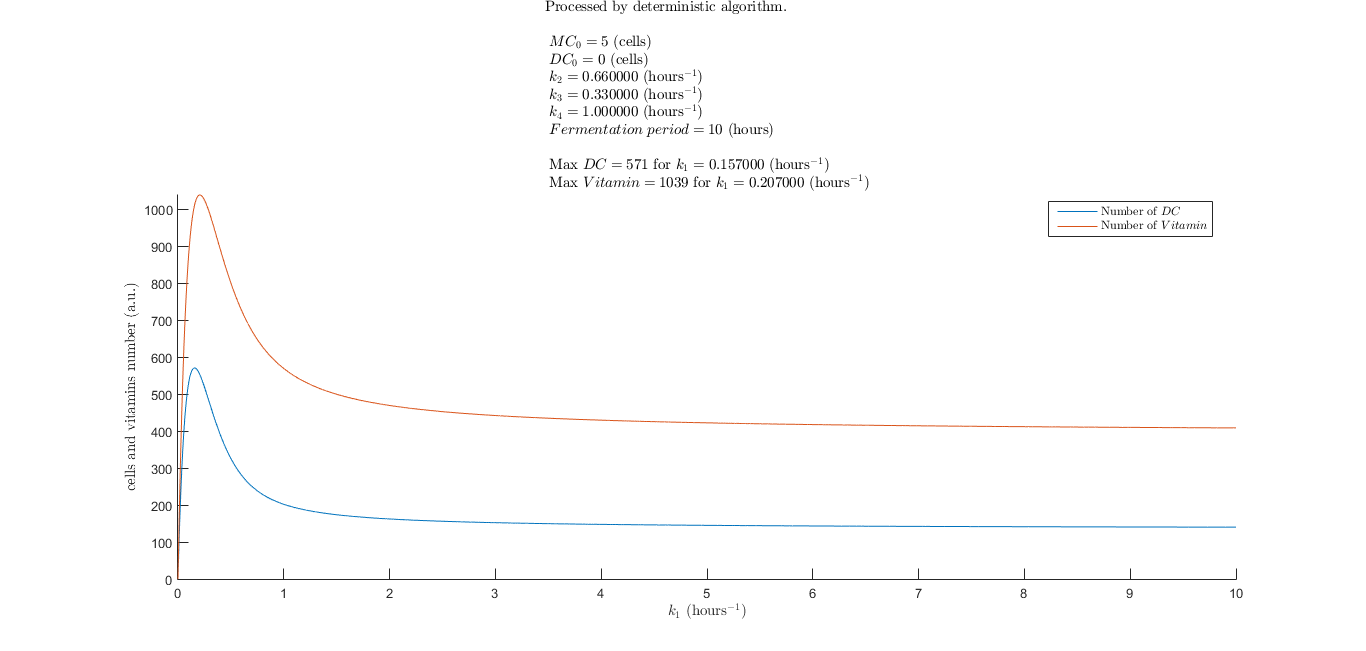Figure 1 : Deterministic vitamin optimization.

As you can see, we are able to find the best $$k_{1}$$ to optimize the vitamin production.
We noticed that two different $$k_{1}$$ exist to optimize the maximum number of differentiated cell $$DC$$ or the maximum number of $$Vitamin$$.
In our case, we chose of course the $$k_{1}$$ that optimizes the vitamin production i.e. $$k_{1} = 0.207 \phantom{t} (hours^{-1})$$.

### Deterministic evolution of mother cell, differentiated cell and vitamin numbers

We wrote a deterministic algorithm with MATLAB using the previous solutions $$(8)$$, $$(9)$$ and $$(10)$$. For those interested, the source code is available on the software section.
In order to optimize the vitamin production, we use the same parameters as previously and set $$k_{1}$$ with the previous resulting value i.e. $$k_{1} = 0.207 \phantom{t} (hours^{-1})$$.
We obtain the following graph.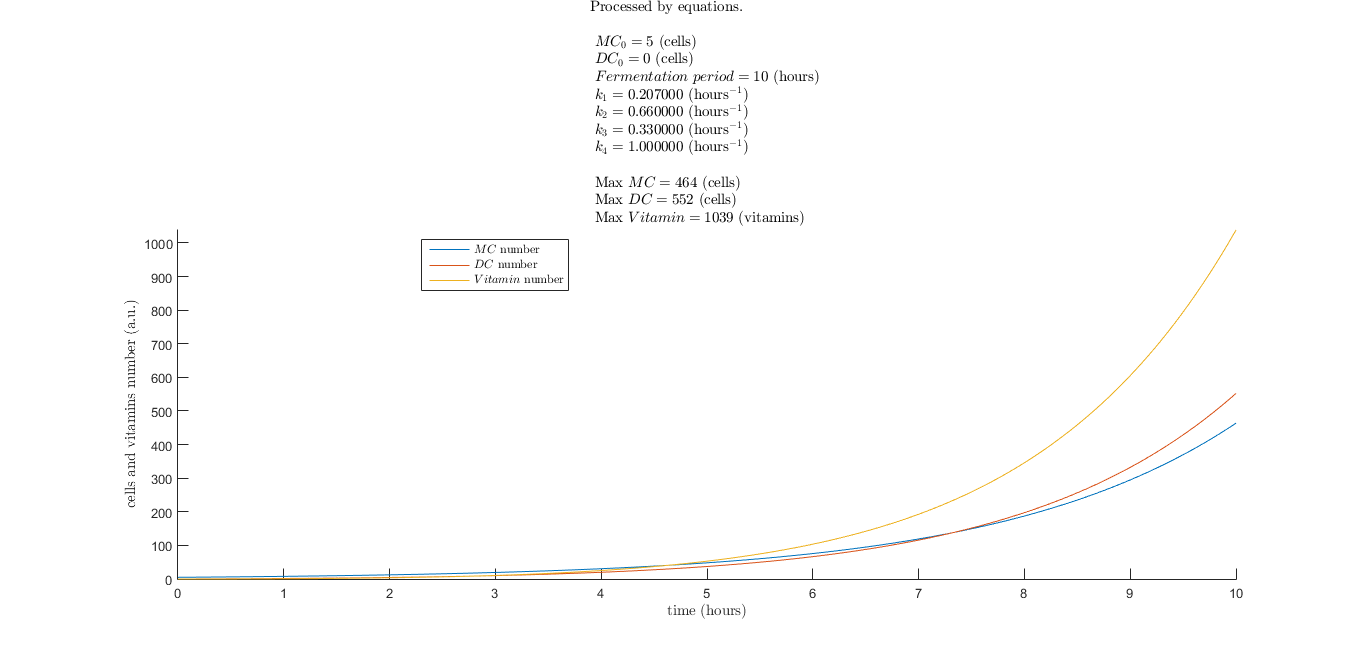Figure 2 : Deterministic evolution of the system.

As you can see, the mother cell, the differentiated cell and the vitamin numbers follow an exponential law of time.
This result seems relevant. The model does not take into account the cells' death and the nutrients present in the medium.

## Stochastic model

### Parameters

We used more parameters than the deterministic model. Indeed, because of the nature of the algorithm, we need a constant rate probability distribution.
In order to do a simulation with laboratory data, we modelize the time probability distribution with a normalized Gaussian distribution using the normrnd MATLAB function.
For each time distribution $$i$$, we use the following time probability distribution. \begin{align} \mathcal P_{i}(t) = \frac{1}{\sigma_{i}.\sqrt{2\pi}}.e^{\frac{-(t - \mu_{i})^2}{2.\sigma_{i}^2}} \end{align} where
• $$\mathcal P_{i}(t)$$ : time $$t$$ probability.
• $$\mu_{i}$$ : time mean.
• $$\sigma_{i}$$ : time standard deviation.

Rate constants for the time distribution $$i$$ are calculated using the following formula. \begin{align} k_{i} = \frac{ln(2)}{t} \end{align} The time $$t$$ is a random variable with a probability $$\mathcal P_{i}(t)$$ as explained above.
The vitamin number is computed by numerical integration using the rate constant $$k_{4}$$.
We have ten parameters for this model.
• $$t$$ : fermentation period.
• $$\mu_{1}$$ : mother cell differentiation time mean.
• $$\sigma_{1}$$ : mother cell differentiation time standard deviation.
• $$\mu_{2}$$ : mother cell doubling time mean.
• $$\sigma_{2}$$ : mother cell doubling time standard deviation.
• $$\mu_{3}$$ : differentiated cell doubling time mean.
• $$\sigma_{3}$$ : differentiated cell doubling time standard deviation.
• $$k_{4}$$ : rate constant of the differentiated cell vitamin production.
• $$MC_0$$ : initial number of mother cells in the medium.
• $$DC_0$$ : initial number of differentiated cells in the medium.

### Algorithm

#### Introduction

In the program, each cell is a structure containing three elements.
• The cell type.
• 1 : mother cell.
• 2 : differentiated cell.
• The next event type.
• 1 : a mother cell becomes a differentiated cell.
• 2 : a mother cell divides into two mother cells.
• 3 : a differentiated cell divides into two differentiated cells.
• The time before the next event.

The time $$T_{i}$$ for an event is chosen thanks to the equation $$(11)$$.
There is a competition between the first two events concerning the mother cell.
Either the mother cell differentiates or it divides into two differentiated cells.
To do the choice we compare $$T_{1}$$ and $$T_{2}$$. The smallest time is the next event time.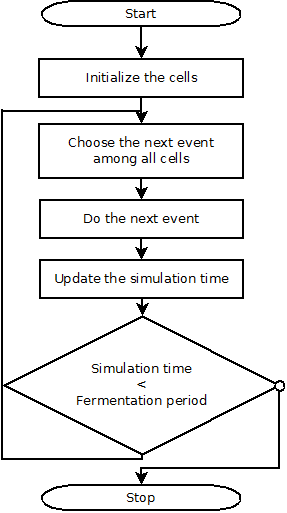#### Step 1 : Initialize the cells.

First of all we have to generate the first cells in accordance with $$MC_0$$ and $$DC_0$$.
For each cell we set the cell type, the next event and the time before the next event.

#### Step 2 : Choose the next event among all cells.

We have an array containing all the cells. Each cell contains the time before its next event (divide or differentiate).
The next event time processed by the algorithm is the smallest cell next event time.

#### Step 3 : Do the next event.

Now that we know the next event type and the concerned cell (only one cell is involved here), we compute this event.
As explained above, we have three choices.
If the cell is a mother cell, the cell can divide or differentiate.
If the cell is a differentiated cell, the cell can only divide.

We define a new event and time event for the cell and for the new cell in case of division.
We also performed the number of vitamin calculus by numerically integrating the number of $$DC$$.
We use the following formula. \begin{align} Vitamin(t) = Vitamin(t-T_{i}) + k_{4}.DC(t).T_{i} \end{align} where
• $$Vitamin(t)$$ : number of vitamin at the instant $$t$$.
• $$DC(t)$$ : number of differentiated cells in the medium at the instant $$t$$.
• $$T_{i}$$ : time of the next event being performed.
• $$k_{4}$$ : rate constant of the differentiated cell vitamin production.

#### Step 4 : Update the simulation time.

For each cell except the concerned cell, define the cell next event time with the following formula. \begin{align} T_{event \phantom{t} cell} = T_{event \phantom{t} cell} - T_{i} \end{align} where
• $$T_{i}$$ : time of the next event being performed.
• $$T_{event \phantom{t} cell}$$ : cell next time event.

#### Step 5 : Go to step 2 until the simulation time is superior to the fermentation period.

We do this process until the simulation time is superior to the fermentation period. The number of cells increases with an exponential law. The computational power increases with the same law.
Do not try to process a long simulation time if your computer is not powerful.

#### Step 6 : Show the results.

Here is a graph generated by the stochastic algorithm.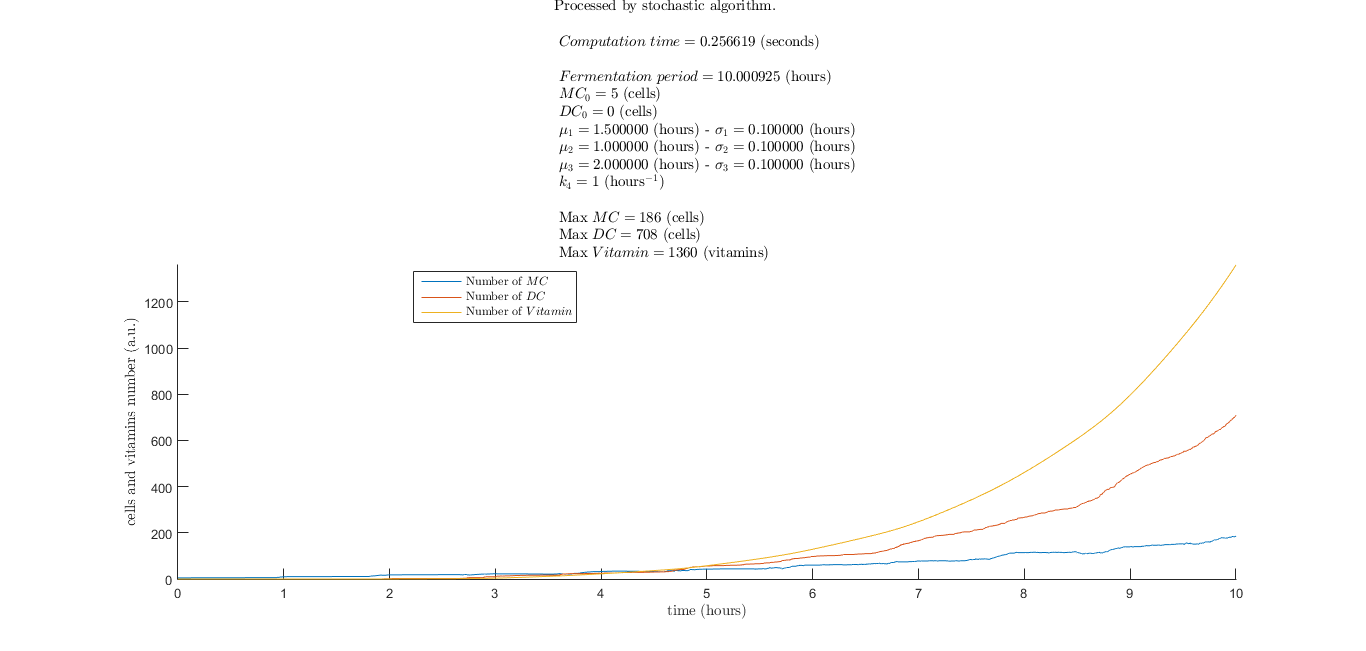Figure 3 : Stochastic evolution of the system.

With the following distribution of event time probability.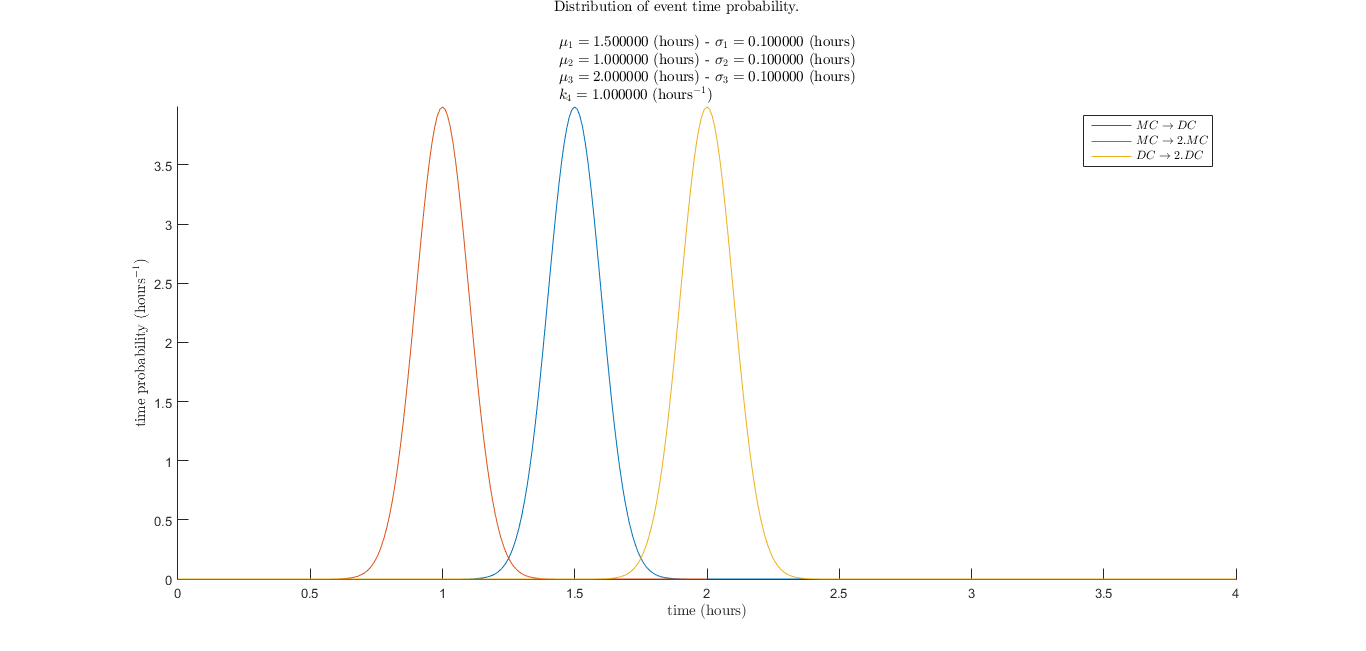Figure 4 : Distribution of event time probability.

### Vitamin optimization

Like the deterministic model our goal is to maximize the vitamin production.
In order to get relevant results, we average by doing a large number of simulations specified by the $$Averaging$$ $$number$$ parameter.
We can only change three parameters : $$\mu_{1}$$, $$MC_0$$ and $$DC_0$$.
$$t$$, $$\sigma_{1}$$, $$\mu_{2}$$, $$\sigma_{2}$$, $$\mu_{3}$$, $$\sigma_{3}$$ and $$k_{4}$$ are constants.
We try to find the best $$\mu_{1}$$ to maximize the vitamin production.
As an example we can consider the following parameters.
• $$t = 5 \phantom{t} (hours)$$
• $$Averaging$$ $$number = 500$$
• $$\sigma_{1} = 0.1 \phantom{t} (hours)$$
• $$\mu_{2} = 1.0502 \phantom{t} (hours)$$
• $$\sigma_{2} = 0.1 \phantom{t} (hours)$$
• $$\mu_{3} = 2.1004 \phantom{t} (hours)$$
• $$\sigma_{3} = 0.1 \phantom{t} (hours)$$
• $$k_{4} = 1 \phantom{t} (hours^{-1})$$
• $$MC_0 = 5 \phantom{t} (cells)$$
• $$DC_0 = 0 \phantom{t} (cells)$$

We obtain the following graph.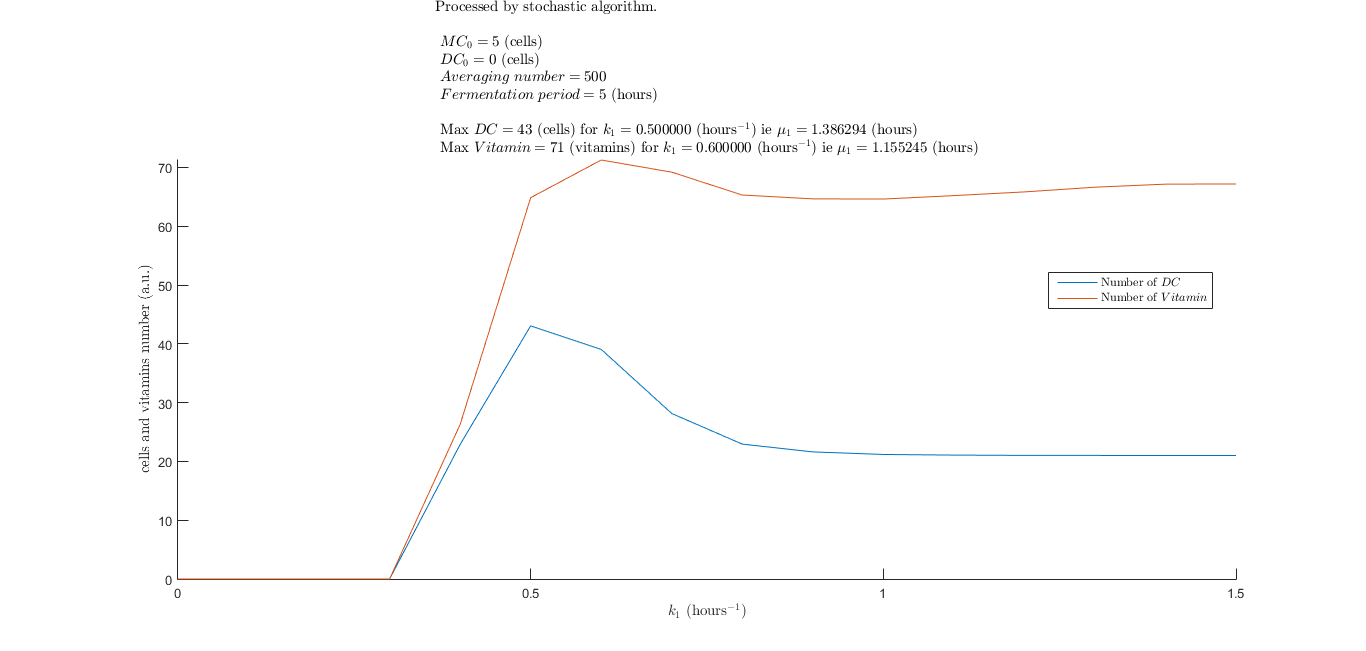Figure 5 : Stochastic vitamin optimization.

Here is a deterministic vitamin optimization.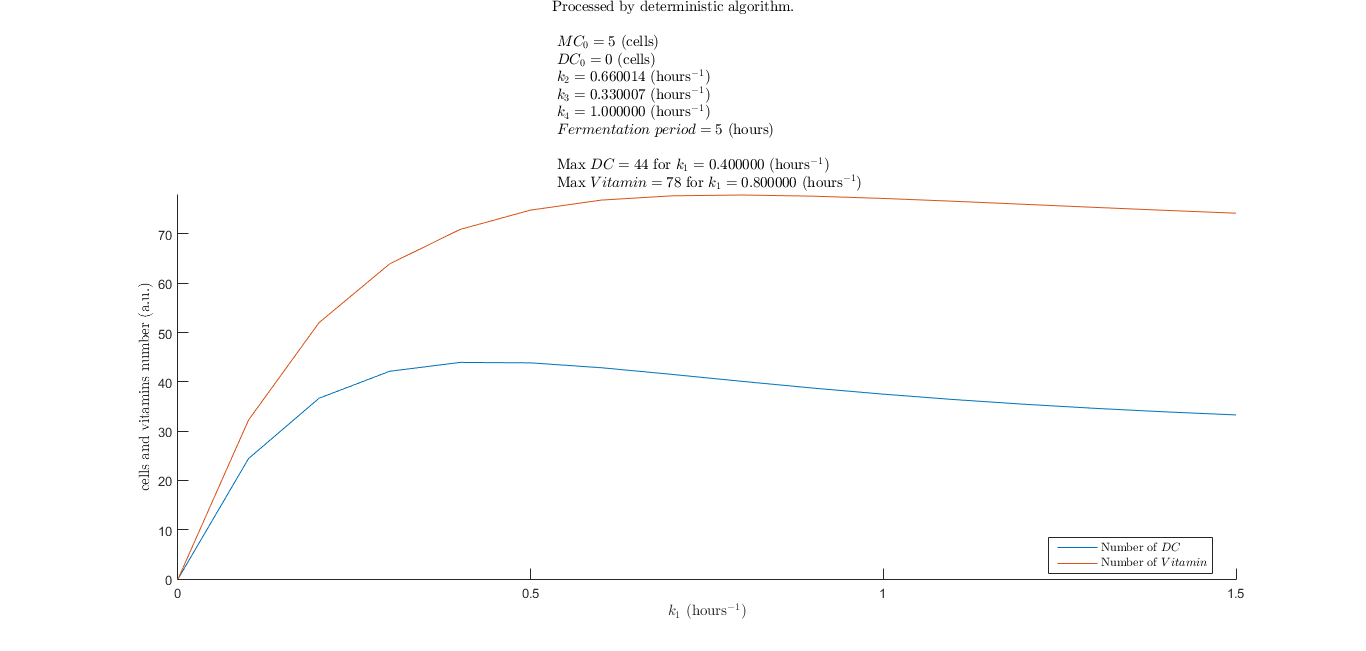Figure 6 : Deterministic vitamin optimization.

We can compare the two results by superimposing the two graphs.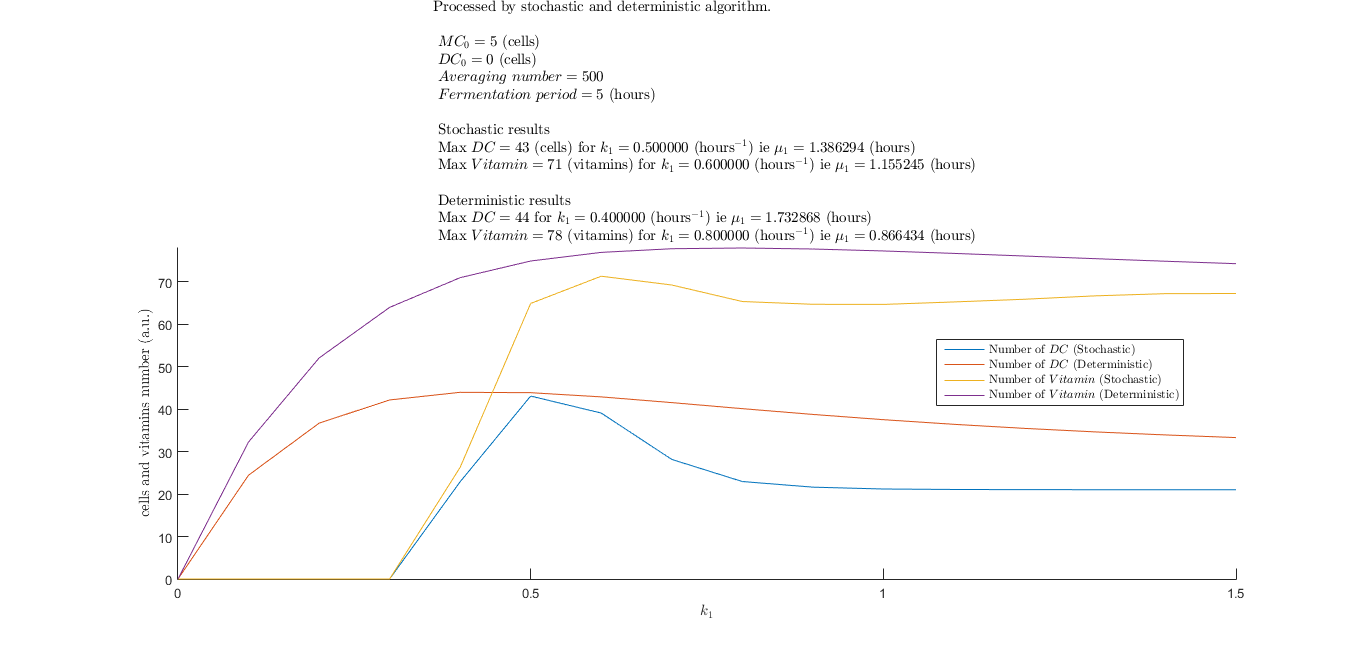Figure 7 : Comparison of deterministic and stochastic vitamin optimization.

We performed a cross-correlation and found correlation coefficients between the two graphs with the xcorr and corr2 MATLAB functions.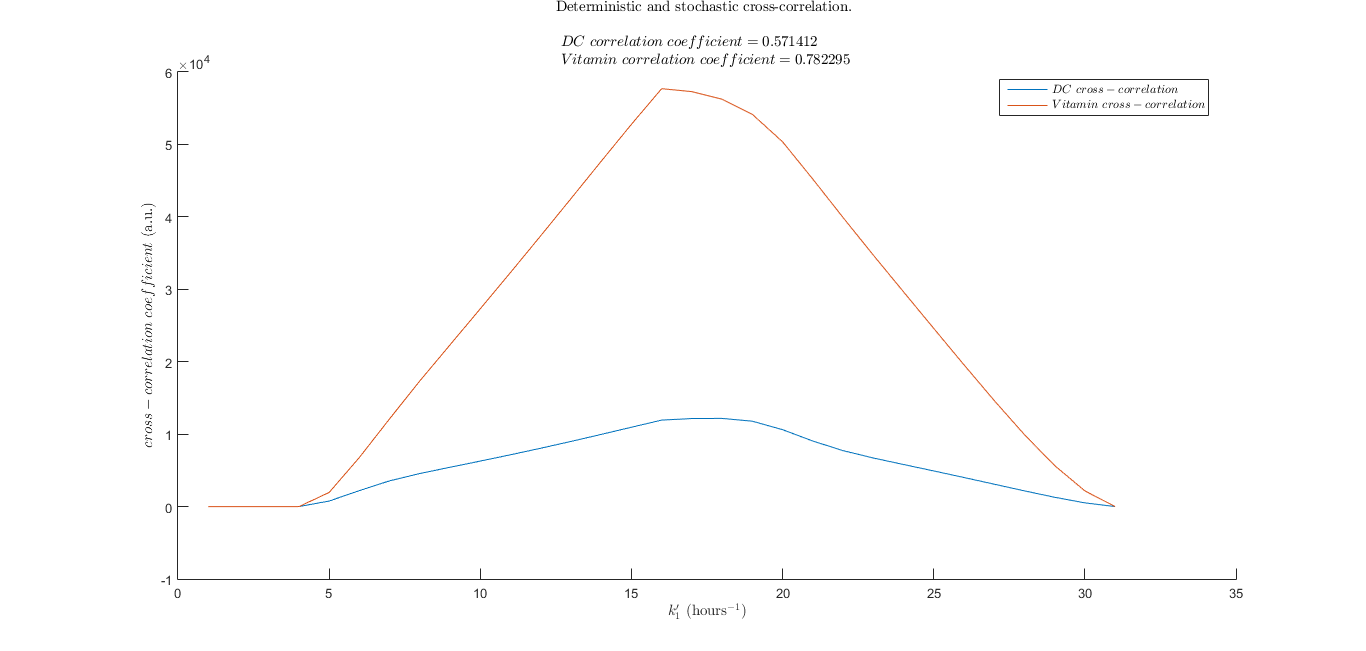Figure 8 : Deterministic and stochastic cross-correlation and correlation coefficients.

As described in the official MATLAB website, the $$DC$$ and $$Vitamin$$ correlation coefficients are computed with the following formula.

\begin{align} DC \phantom{t} correlation \phantom{t} coefficient = \frac{\sum\limits^{}_{k_{1}} (DC_{d}(k_{1}) - \overline{DC_{d}}).(DC_{s}(k_{1}) - \overline{DC_{s}})} {\sqrt{\left(\sum\limits^{}_{k_{1}} (DC_{d}(k_{1}) - \overline{DC_{d}})^2 \right).\left(\sum\limits^{}_{k_{1}} (DC_{s}(k_{1}) - \overline{DC_{s}})^2 \right)}} \end{align}
\begin{align} Vitamin \phantom{t} correlation \phantom{t} coefficient = \frac{\sum\limits^{}_{k_{1}} (Vitamin_{d}(k_{1}) - \overline{Vitamin_{d}}).(Vitamin_{s}(k_{1}) - \overline{Vitamin_{s}})} {\sqrt{\left(\sum\limits^{}_{k_{1}} (Vitamin_{d}(k_{1}) - \overline{Vitamin_{d}})^2 \right).\left(\sum\limits^{}_{k_{1}} (Vitamin_{s}(k_{1}) - \overline{Vitamin_{s}})^2 \right)}} \end{align}
The $$DC$$ and $$Vitamin$$ cross-correlation are computed with these equations.

\begin{align} DC \phantom{t} cross\text{-}correlation(k_{1}') = \sum\limits^{}_{k_{1}} DC_{d}(k_{1}).DC_{s}(k_{1} + k_{1}') \end{align} \begin{align} Vitamin \phantom{t} cross\text{-}correlation(k_{1}') = \sum\limits^{}_{k_{1}} Vitamin_{d}(k_{1}).Vitamin_{s}(k_{1} + k_{1}') \end{align} where
• $$DC_{d}(k_{1})$$ : deterministic $$DC$$ function of $$k_{1}$$.
• $$DC_{s}(k_{1})$$ : stochastic $$DC$$ function of $$k_{1}$$.
• $$Vitamin_{d}(k_{1})$$ : deterministic $$Vitamin$$ function of $$k_{1}$$.
• $$Vitamin_{s}(k_{1})$$ : stochastic $$Vitamin$$ function of $$k_{1}$$.

## Conclusion

As we can see, the stochastic and the deterministic models provide a priori different results. It seems logical because of the statistical nature of the problem.
However, the comparison of the two graphs gives us useful and accurate information about the vitamin optimization.
Because of small cell counts, the stochastic and discrete method has a significant influence on the observed behaviour.
In vivo, the cell population likely follows the stochastic model. We are waiting for laboratory results to confirm or disprove our model.
We are able to maximize the vitamin production by determining the best rate constant $$k_{1}$$ and therefore the best doubling time $$\mu_{1}$$ to set the differentiation rate.

# Software

## Introduction

As explained in the modeling section, this program models the cell population evolution with mother cell differentiation and cell division.
In order to make this model and code accessible, understandable and editable by everyone, we have created this software section.
We focus here on the stochastic program.

## Files

The MATLAB code is available on GitHub under the GNU General Public License version 3.0.
Feel free to download the source code, modify it and make it accessible for everyone.
Here is a quick explanation of the different files i.e. the different functions.

### Stochastic algorithm

• $$timeEvolutionStochastic$$ : calculate the time evolution.
• $$checkInput$$ : check the input and show an error message if the input is not good.
• $$elmPixel$$ : find the coordinates of a pixel around another pixel.
• $$errorProgramm$$ : display an error and stop the program.
• $$freePosition$$ : create a list of free pixels around one pixel.
• $$getComputerName$$ : get the computer name.
• $$getSizeMat$$ : find the matrix size.
• $$imageToMatrix$$ : convert an image into a matrix.
• $$initCells$$ : initialize the cells.
• $$initialization$$ : initialize the program.
• $$initTimeEvent$$ : calculate the different time.
• $$initVariables$$ : initialize the variables.
• $$isBinary$$ : check if a number is 0 or 1.
• $$isPositiveInteger$$ : check if a number is a positive integer (1) or not (0).
• $$makeCells$$ : make the cells.
• $$randEvent$$ : generate the next random event.
• $$randPosition$$ : find a free position for a cell.
• $$saveInitialParameters$$ : save the initial parameters in a text file.
• $$saveResult$$ : save and plot the results.
• $$showAndSaveEventTimeDistribution$$ : show and save the event time probability distribution.
• $$showPopulation$$ : show the cells matrix.
• $$writeParameters$$ : write a text file with the parameters.
• $$launchStochasticProgramm$$ : launch the stochastic time evolution simulation.
• $$k1OptimizationStochastic$$ : calculate the number of differentiated cells and vitamin with different $$k_{1}$$ in a stochastic way.
• $$k1OptimizationStochasticAndDeterministic$$ : superimpose the deterministic and stochastic models.

### Deterministic algorithm

• $$timeEvolutionDeterministic$$ : show the time evolution by numerical calculus and equations.
• $$k1OptimizationDeterministic$$ : calculate the number of differentiated cells with different $$k_{1}$$ in a deterministic way.

## Input

In order to launch a simulation, you must define five variables.
• Initial cells.
• Rate constants.
• Fermentation period.
• Action.
• Folder name.

### Cells input

You can choose to generate a random or a predetermined position for the initial cells.
You have two cell types : mother cells and differentiated cells.

#### Random initial position

The input array must contain four integer values in this order.
• $$MC_0$$ : initial number of mother cells in the medium.
• $$DC_0$$ : initial number of differentiated cells in the medium.
• $$sizeMatCell$$ : cells box size (in pixels).
• $$sizeMat$$ : real box size (in pixels).

Warning : $$sizeMatCell$$ must be larger than $$sizeMat$$.

In the program, the input variable has the following pattern.
$$input = [MC_0, DC_0, sizeMatCell, sizeMat]$$

Here is an example.
• $$MC_0 = 5 \phantom{t} (cells)$$
• $$DC_0 = 0 \phantom{t} (cells)$$
• $$sizeMatCell = 100 \phantom{t} (pixels)$$
• $$sizeMat = 250 \phantom{t} (pixels)$$

#### Predetermined initial position

In order to make an easy predetermined initial position input, we wrote a code that understands images.
The input must contain the image path.
It is very easy to design a custom image i.e. a custom spatial cell distribution. You must follow these four rules (we work only with the image red component)
• the image must be square and in jpeg format.
• 255 means no cell.
• 128 means one differentiated cell.
• 0 means one mother cell.
0, 128 and 255 are red components of any colours that you want because the MATLAB code take into account only the image red component.
If you want, you can use the following hexadecimal colors codes.
• #FFFFFF (white) means no cell.
• #800000 (red) means one differentiated cell.
• #000000 (black) means one mother cell.

Here is an example only with mother cells.Figure 9 : Example of predetermined cells initial position with mother cells.

Here is another example with mother cells (black) and differentiated cells (red).Figure 10 : Example of predetermined cells initial position with mother cells (black) and differentiated cells (red).

### Rate constants

You have to define seven variables.
• $$\mu_{1}$$ : mother cell differentiation time mean.
• $$\sigma_{1}$$ : mother cell differentiation time standard deviation.
• $$\mu_{2}$$ : mother cell doubling time mean.
• $$\sigma_{2}$$ : mother cell doubling time standard deviation.
• $$\mu_{3}$$ : differentiated cell doubling time mean.
• $$\sigma_{3}$$ : differentiated cell doubling time standard deviation.
• $$k_{4}$$ : rate constant of the differentiated cell vitamin production.

In the program, the rate constants variable has the following pattern.
$$constantRate = [\mu_{1}, \sigma_{1}, \mu_{2}, \sigma_{2}, \mu_{3}, \sigma_{3}, k_{4}]$$

Here is an example.
• $$\mu_{1} = 1 \phantom{t} (hours)$$
• $$\sigma_{1} = 0.1 \phantom{t} (hours)$$
• $$\mu_{2} = 1.0502 \phantom{t} (hours)$$
• $$\sigma_{2} = 0.1 \phantom{t} (hours)$$
• $$\mu_{3} = 2.1004 \phantom{t} (hours)$$
• $$\sigma_{3} = 0.1 \phantom{t} (hours)$$
• $$k_{4} = 1 \phantom{t} (hours^{-1})$$

### Fermentation period

The fermentation period $$t$$ is a simple scalar variable.
You can use $$t = 5 \phantom{t} (hours)$$ for example.

### Action

In order to choose what you want to do, you must define three Boolean variables.
• $$createFolder$$ : create a folder in the disk to save information.
• $$showAnimation$$ : show the cell population animation. Warning : requires a lot of computing power.
• $$plotGraph$$ : plot the results in graphs.

In the program, the action variable has the following pattern.
$$action = [createFolder, showAnimation, plotGraph$$]

In the following example we want to create a folder and plot the graphs.
• $$createFolder = 1$$
• $$showAnimation = 0$$
• $$plotGraph = 1$$

In the following example we want to show the cell animation.
• $$createFolder = 0$$
• $$showAnimation = 1$$
• $$plotGraph = 0$$

In the following example we want to show the cell animation and save the images in a folder.
With the images you can create gif animations.
• $$createFolder = 1$$
• $$showAnimation = 1$$
• $$plotGraph = 0$$

### Folder name

The $$folderName$$ variable is a string containing the folder name where results folder are stored in the disk.
You can use $$folderName =$$ '$$Results$$' for example.

## Output

Four types of output are generated.
• raw data : all the useful information.
• folder creation : if chosen in the $$action$$ variable a folder is created.
• graphs : if chosen in the $$action$$ variable graphs are generated.
• animation : if chosen in the $$action$$ variable cell animation is generated.

### Raw data

• $$mainTic$$ : start of the program.
• $$MC_0$$ : initial number of mother cells in the medium.
• $$DC_0$$ : initial number of differentiated cells in the medium.
• $$pasDistributionTimeEvent$$ : resolution for the graph time event distribution plot (see the $$initVariables$$ function).
• $$frameRate$$ : animation frame rate (see the $$initVariables$$ function).
• $$timeShowPopulation$$ : simulation time between two animations (see the $$initVariables$$ function).
• $$simulationTime$$ : fermentation period $$t$$.
• $$resultFolderName$$ : folder where the results folder are created.
• $$\mu_{1}$$ : mother cell differentiation time mean.
• $$\sigma_{1}$$ : mother cell differentiation time standard deviation.
• $$\mu_{2}$$ : mother cell doubling time mean.
• $$\sigma_{2}$$ : mother cell doubling time standard deviation.
• $$\mu_{3}$$ : differentiated cell doubling time mean.
• $$\sigma_{3}$$ : differentiated cell doubling time standard deviation.
• $$k_{4}$$ : rate constant of the differentiated cell vitamin production.
• $$cVitamin$$ : vitamin counter.
• $$time$$ : main timer containing the simulation time.
• $$createFolder$$ : action boolean.
• $$showPopulationBool$$ : action boolean.
• $$plotGraph$$ : action boolean.
• $$input$$ : input array.
• $$cTime1$$ : time distribution counter 1 (see the $$randEvent$$ function).
• $$cTime2$$ : time distribution counter 2 (see the $$randEvent$$ function).
• $$cTime3$$ : time distribution counter 3 (see the $$randEvent$$ function).
• $$counterEvent$$ : event counter.
• $$timerShow$$ : time animation counter.
• $$sizeMat$$ : real box size (in pixels).
• $$nextEvent$$ : next event type.
• $$time1Array$$ : time distribution array 1 (see the $$initTimeEvent$$ function).
• $$time2Array$$ : time distribution array 2 (see the $$initTimeEvent$$ function).
• $$time3Array$$ : time distribution array 3 (see the $$initTimeEvent$$ function).
• $$cell$$ : structure containing all the cells' information.
• $$sizeMatCell$$ : cells box size (in pixels).
• $$timeNextEvent$$ : time before the next event.
• $$saveTime$$ : array containing the simulation time evolution.
• $$saveNbrMC$$ : array containing the mother cells time evolution.
• $$saveNbrDC$$ : array containing the differentiated cells time evolution.
• $$saveNbrVitamin$$ : array containing the vitamin time evolution.
• $$folderName$$ : folder where the results are stored. The pattern is the following : $$resultFolderName/DD-MM-YY\_hh\_mm\_ss\_mss$$.
• $$imgType$$ : image type (see the $$initialization$$ function).
• $$parametersFileName$$ : parameters file name where the parameters are saved (see the $$initialization$$ function).
• $$parametersDoc$$ : file object where the parameters are saved.
• $$maxMC$$ : maximum number of mother cells.
• $$maxDC$$ : maximum number of differentiated cells.
• $$maxVitamin$$ : maximum number of vitamins.
• $$timeSpent$$ : computation time (in seconds).

### Folder creation

If the $$action$$ variable $$createFolder$$ is set, the program will save important results in this folder.
• $$parameters.txt$$ : text file containing different simulation parameters and results.
• $$data.mat$$ : MATLAB file containing the raw data structure. To load the file, use this formula : $$load('data.mat')$$.

### Graphs

If the $$action$$ variable $$plotGraph$$ is set, the program will show the graphs.
Moreover, if the $$action$$ variable $$createFolder$$ is set, the program will save the graphs in the folder.

### Animation

If the $$action$$ variable $$showAnimation$$ is set, the program will show the cell animation.
Moreover, if the $$action$$ variable $$createFolder$$ is set, the program will save the images in the folder.

## Examples

We present here some results obtained with the program. For more details about the model sees the modeling section.

### Time evolution of mother cells, differentiated cells and vitamin

#### Graphs

As explained in the modeling section the program give these results.Figure 11 : Stochastic evolution of the system.Figure 12 : Stochastic vitamin optimization.Figure 13 : Distribution of event time probability.

#### Animation

Here is an example with mother cells (black) and differentiated cells (red).
This image is the cell input.Figure 14 : Example of predetermined cells initial position with mother cells (black) and differentiated cells (red).

The result is the following animation after a conversion in a gif file.
The mother cells are in orange and the differentiated cells are in yellow.Figure 15 : Animation example with predetermined cells initial position with mother cells and differentiated cells.

### Vitamin optimization

This program is designed to maximize the vitamin production. Here are some results.Figure 16 : Stochastic vitamin optimization.

### Deterministic and stochastic vitamin optimization comparison

Here is a comparison between the two models.Figure 17 : Comparison of deterministic and stochastic vitamin optimization.

## Conclusion

Feel free to use and modify this program. The source code is available on GitHub : iGEM Paris Bettencourt 2015 under the GNU General Public License version 3.0. For more information concerning the model see the modeling section.